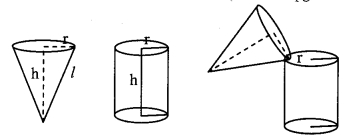# Maharashtra Board 9th Class Maths Part 2 Practice Set 9 Solutions Chapter 9 Surface Area and Volume

Balbharti Maharashtra State Board Class 9 Maths Solutions covers the Practice Set 9 Geometry 9th Class Maths Part 2 Answers Solutions Chapter 9 Surface Area and Volume.

## Practice Set 9 Geometry 9th Std Maths Part 2 Answers Chapter 9 Surface Area and Volume

Question 1.
If diameter of a road roller is 0.9 m and its length is 1.4 m, how much area of a field will be pressed in its 500 rotations? ( π = $$\frac { 22 }{ 7 }$$)
diameter (d) = 0.9 m, length (h) = 1.4 m
To find: Area of a field pressed in 500 rotations
Solution:
i. Since, area of field pressed in 1 rotation of road roller = curved surface area of road roller
∴ Curved surface area of the road roller = 2πrh
= πdh ,..[∵ d = 2r]
= $$\frac { 22 }{ 7 }$$ x 0.9 x 1.4 7
= 22 x 0.9 x 0.2
= 3.96 sq.m.

ii. Area of land pressed in 1 rotation = 3.96 sq.m.
∴Area of land pressed in 500 rotations = 500 x 3.96
= 1980 sq.m.
∴ 1980 sq.m, land will be pressed in 500 rotations of the road roller.

Question 2.
To make an open fish tank, a glass sheet of 2 mm gauge is used. The outer length, breadth and height of the tank are 60.4 cm, 40.4 cm and 40.2 cm respectively. How much maximum volume of water will be contained in it ?
Given: Thickness of the glass = 2 mm,
outer length of the tank = 60.4 cm,
outer breadth of the tank = 40.4 cm,
outer height of the tank = 40.2 cm
To find: Volume of water fish tank contains
Solution:i. Thickness oldie glass = 2 mm.
= $$\frac { 2 }{ 10 }$$ cm
= 0.2 cm
Outerlengthofthetank = 60.4 cm
∴ Inner length oldie tank (l) = Outer length – thickness oldie glass on both sides
= 60.4 – 0.2 – 0.2
= 60cm
Outer breadth oldie tank = 40.4 cm
∴ Inner breadth of the tank (b) = 40.4 – 0.2 – 0.2
= 40 cm
Outer height of the tank = 40.2 cm
∴Inner height of the tank (h) = 40.2 – 0.2
= 40 cm

ii. Maximum volume of water that can be contained in the tank = volume of the tank
= l x b x h
= 60 x 40 x 40
= 96000 cubic cm.
∴ The fishtank can contain maximum of 96000 cubic cm. water in it.

Question 3.
If the ratio of radius of base and height of a cone is 5 : 12 and its volume is 314 cubic metre. Find its perpendicular height and slant height (π = 3.14).
Given: Ratio of radius of base and height of a cone = 5 : 12,
Volume = 314 cubic metre
To find: Perpendicular height (h) and slant height (l)
Solution:
i. The ratio of radius and height of cone is 5 : 12
Let the common multiple be x.
∴ Radius of base (r) = 5x
Perpendicular height (h) = 12x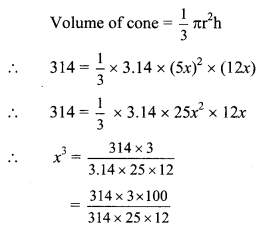∴ x3 = 1
∴ x = 1 … [Taking cube root on both sides]
∴ r = 5x = 5(1) = 5m
h = 12x = 12(1) = 12 m

ii. Now, l2 = r2 + h2
= 52 + 122
= 25 + 144
∴l2 = 169
∴ l = $$\sqrt { 169 }$$ … [Taking square root on both sides]
= 13 m
The perpendicular height and slant height of the cone are 12 m and 13 m respectively.

Question 4.
Find the radius of a sphere if its volume is 904.32 cubic cm. (π = 3.14)
Given: Volume of sphere = 904.32 cubic cm.
To find: Radius of a sphere
Solution:
Volume of sphere = $$\frac { 4 }{ 3 }$$ πr3
∴ 904.32 = $$\frac { 4 }{ 3 }$$ x 3.14 x r3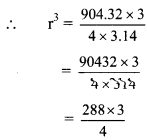= 216
∴ r = $$\sqrt [ 3 ]{ 216 }$$ … [Taking cube root on both sides]
= 6 cm
∴ The radius of the sphere is 6 cm.

Question 5.
Total surface area of a cube is 864 sq.cm. Find its volume.
Given: Total surface area of cube = 864 sq. cm
To find: Volume of cube
Solution:
i. Total surface area of cube = 6l2
∴ 864 = 6l2
∴ l2= $$\sqrt [ 864 ]{ 6 }$$
∴ l2 = 144
∴ l = $$\sqrt { 144 }$$ … [Taking square root on both sides]
= 12 cm

ii. Volume of cube = l2
= 123
= 1728 cubic cm.
∴ The volume of cube is 1728 cubic cm.

Question 6.
Find the volume of a sphere, if its surface area is 154 sq.cm.
Given: Surface area of sphere = 154 sq. cm.
To find: Volume of sphere
Solution:
i. Surface area of sphere = 4πr2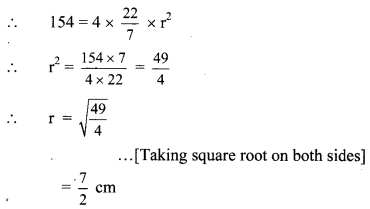∴ The volume of sphere is 179.67 cubic cm.

Question 7.
Total surface area of a cone is 616 sq.cm. If the slant ‘height of the cone Is three times the radius of its base, find its slant height.
Given: Total surface area of a cone = 616 sq.cm., slant height of the cone is three times the radius of its base
To find: Slant height (l)
Solution:
i. Let the radius of base be r cm.
∴ Slant height (l) = 3r cm
Total surface area of cone = πr (l + r)
∴ 616 = πr(l + r)
∴ 616 = $$\sqrt [ 22 ]{ 7 }$$ x r x (3r + r)
∴ 616 = $$\sqrt [ 22 ]{ 7 }$$ x 4r2∴ r2 = 49
∴ r = $$\sqrt { 49 }$$ … [Taking square root on both sides]
= 7

ii. Slant height (l) = 3r = 3 x 7 = 21 cm
∴ The slant height of the cone is 21 cm.

Question 8.
The inner diameter of a well is 4.20 metre and its depth is 10 metre. Find the inner surface area of the well. Find the cost of plastering it from inside at the rate ₹ 52 per sq.m.
Given: Inner diameter (d) = 4.2 m,
To find: depth (h) = 10 m,
rate of plastering = ₹ 52 per sq.m.
Inner surface area and total cost of plastering
Solution:
i. Inner curved surface area of the well = 2πrh
= πdh …[∵ d = 2r]
= $$\sqrt [ 22 ]{ 7 }$$ x 4.2 x 10
= $$\sqrt [ 22 ]{ 7 }$$ x 42
= 22 x 6
= 132 sq.m.

ii. Rate of plastering = ₹52 per sq.m.
∴ Total cost = Curved surface area x Rate of plastering
= 132 x 52 = ₹6864
∴ The cost of plastering the well from inside is ₹6864.

Question 9.
The length of a road roller is 2.1 m and its diameter is 1.4 m. For levelling a ground 500 rotations of the road roller were required. How much area of ground was levelled by the road roller? Find the cost of levelling at the rate of ₹ 7 per sq.m.
diameter (d) = 1.4 m,
length (h) = 2.1 m
number of rotations required for levelling the ground = 500,
rate of levelling = ₹ 7 per sq. m.
To find: Area of ground leveled by the road roller and cost of levelling
Solution:
i. Since, area of ground levelled in 1 rotation of road roller = curved surface area of road roller
∴Curved surface area of the road roller = 2πrh
= πdh …[∵ d = 2r]
= $$\frac { 22 }{ 7 }$$ x 1.4 x 2.1
= 22 x 0.2 x 2.1
= 9.24 sq.m.

ii. Area of ground levelled in 1 rotation = 9.24 sq.m.
∴Area of ground levelled in 500 rotations = 9.24 x 500
= 4620 sq.m.

iii. Rate of levelling ₹ 7 per sq.m.
∴Total cost = Area of ground levelled x Rate of levelling
= 4620 x 7
= ₹32340
∴ The road roller levels 4620 sq.m. land in 500 rotation, and the cost of levelling is ₹32340.

Maharashtra Board Class 9 Maths Chapter 9 Surface Area and Volume Practice Set 9 Intext Questions and Activities

Question 1.
Curved surface area of cone. (Textbook pg. no. 116)Circumference of base of the cone = 2πr
As shown in the figure (c), make pieces of the net as small as possible. Join them as shown in the figure (d),. By joining the small pieces of net of the cone, we get a rectangle ABCD approximately.
Total length of AB and CD is 2πr.
∴ length of side AB of rectangle ABCD is πr and length of side CD is also πr.
Length of side BC of rectangle = slant height of cone = l.
Curved surface area of cone is equal to the area of the rectangle.
∴ curved surface area of cone = Area of rectangle = AB x BC = πr x l = πrl

Question 2.
Prepare a cylinder of a card sheet, keeping one of its faces open. Prepare an open cone of card sheet which will have the same base-radius and the same height as that of the cylinder. Pour fine sand in the cone till it just fills up the cone. Empty the cone in the cylinder. Repeat the procedure till the cylinder is just filled up with sand. Note how many coneful of sand is required to fill up the cylinder. (Textbook pg, no 117)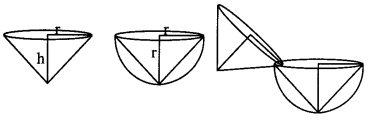To fill the cylinder, three coneful of sand is required.

Question 3.
Finding total surface area of sphere. (Textbook pg, no 120)

i. Take a sweet lime (Mosambe), Cut it into two equal parts.ii. Take one of the parts. Place its circular face on a paper. Draw its circular border. Copy three more such circles. Again, cut each half of the sweet lime into two equal parts.iii. Now you get 4 quarters of sweet lime. Separate the peel of a quarter part. Cut it into pieces as small as possible. Try to cover one o’f the circles drawn, by the small pieces. Observe that the circle gets nearly covered.
The activity suggests that,
Curved surface area of a sphere = 4πr2∴ Curved surface area of a sphere = 4 x Area of a circle

Question 4.
Make a cone and a hemisphere of cardsheet such that radii of cone and hemisphere are equal and height of cone is equal to radius of the hemisphere.
Fill the cone with fine sand. Pour the sand in the hemisphere. How many cones are required to fill the hemisphere completely ? (Textbook pg. no. 121)